# What are angles and their types?

• Last Updated : 22 Sep, 2021

Branch of mathematics that deals with angles, measurements, lines are called Geometry. Angles are one of the most important topics in Geometry. When two lines intersect at some point they will be aligned at some magnitude which can be measured using angles. Angulus is a Latin word that means corner from which Angle is derived. The angle can also be formed between two curved lines. The angle between two lines can be represented using the symbol ‘∠’. Angles are measured using degrees and the tool that measures angles is called a protractor.

### What are angles and their types?

The angle can be defined as the amount of rotation between two divergent rays or lines that come from the same point. When two lines intersect they will be aligned at some angle which is measured using degrees. Figure 1 shows the angle ∠ACB.

Attention reader! All those who say programming isn't for kids, just haven't met the right mentors yet. Join the  Demo Class for First Step to Coding Coursespecifically designed for students of class 8 to 12.

The students will get to learn more about the world of programming in these free classes which will definitely help them in making a wise career choice in the future.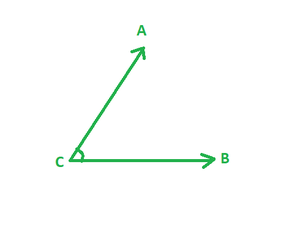Figure: 1 Angle

Different Parts of Angle

An angle comprises of three parts, they are:

• Vertex ⇢ The common point between two rays where an angle is formed is called a vertex. In Figure 1 of Angle ∠ACB point, C is called a vertex.
• Arms or Sides ⇢ The two lines that intersect to form an angle are called Arms or sides of an angle. In figure 1 of Angle ∠ACB lines, AC and CB are called sides or arms.
• Angle ⇢ The measure of rotation of arms or sides along the vertex is called Angle.

### Classification of Angles

Angles are divides based on two factors,

1. Based on the measure of angle or magnitude of the angle.
2. Based on the type of Rotation.

Based on the measure, the angles are divided into 7 main types. These are:

Zero Angle

Zero angles are defined as an angle whose magnitude is 00. It is formed when two arms that intersect to form an angle, lie at the same position. In the figure below lines are diverging from the same point and in the same direction, the angle formed between them is Zero angle.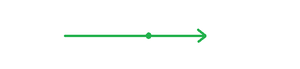Zero Angle

Acute Angle

Acute angle is an angle whose magnitude is greater than 0° and less than 90° is called an Acute angle. The figure below illustrates acute angle ∠ACB between arms AC and CB. Examples: 45°, 57°, 89°Acute Angle

Right Angle

The right angle is an angle whose magnitude is exactly equal to 90° is called a Right angle. The figure below illustrates Right angle ∠ACB between arms AC and CB.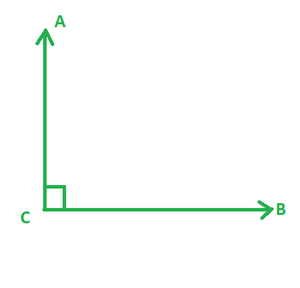Right Angle

Obtuse Angle

Obtuse angle is an angle whose magnitude is greater than 90° and less than 180° is called an Obtuse angle. The figure below illustrates obtuse angle ∠ACB between arms AC and CB.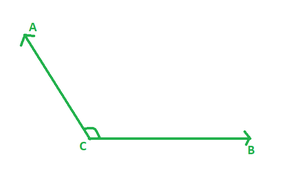Obtuse Angle

Straight Angle

A straight angle is an angle whose magnitude is exactly equal to 180° is called a Straight angle. The figure below illustrates Straight angle ∠ACB between arms AC and CB.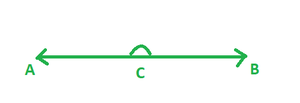Straight Angle

Reflex Angle

Reflex angle is an angle whose magnitude is greater than 180° and less than 360° is called a Reflex angle. The figure below illustrates Reflex angle ∠ACB between arms AC and CB.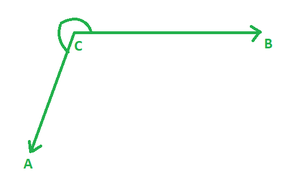Reflex Angle

Complete Angle or Full Rotation Angle

Complete angle is defined as an angle whose magnitude is 360°. It is formed when one of the two arms makes a complete 360 degrees rotation to form an angle. The figure below illustrates the Complete Angle.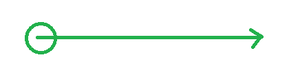Complete Angle

Based on the type of Rotation the angles are divided into two types,

Positive Angles

When the measurement of the angle between two arms is done in anti-clock or counter clock direction from the base then these angles are called Positive Angles.

Negative Angles

When the measurement of the angle between two arms is done in a clockwise direction from the base then these angles are called Negative Angles. Now, let’s take a look at some types that are based on forming a right angle, a straight line, etc.

Complementary Angles

When a pair of angles whose sum is equal to 90° then the pair of angles are said to be Complementary angles. In the figure below the sum of angles ∠ACD and ∠DCB is equal to 90°. So these angles are said to be Complementary angles.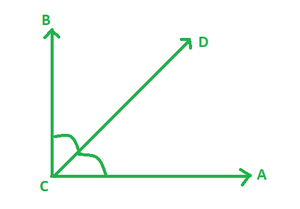Complementary Angles

Supplementary Angles

When a pair of angles whose sum is equal to 180° then the pair of angles are said to be Supplementary angles. In the figure below the sum of angles ∠ACD and ∠DCB is equal to 180°. So these angles are said to be Supplementary angles.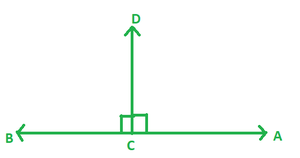Supplementary Angles

### Sample Questions

Question 1: Find the complement of angle 45°?

Solution:

Given,

Angle = 45°

Since complementary angles sum to 90°

Given angle + complement angle = 90°

45 + x = 90

complement angle = 90 – 45 = 45°

Therefore, Complement angle is 45°

Question 2: What is meant by Right angle?

The angle whose measure is 90° then that angle is called as Right angle. The line segments that are joined together

to form Right angle are called as perpendiculars.

Question 3: The angle between two line segments is 310° what kind of angle is this?

Since the angle measured is greater than 180° and less than 360° it is said to be a Reflex angle.

Question 4: Find the supplement of angle 45°?

Solution:

Given,

Angle = 45°

Since sum of supplemetary angles is 180°

Given angle + supplement angle = 180°

45 + x = 180

Supplement angle = 180 – 45 = 135°

Therefore, supplement angle is 135°

Question 5: Angle between two line segments is 10° what kind of angle is this?